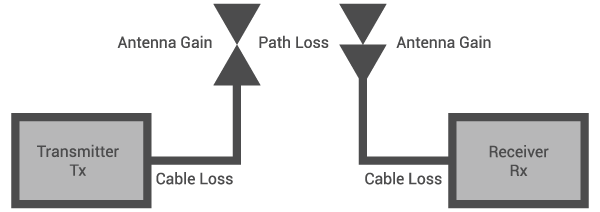# Free Space Path Loss Calculator

## This calculator computes for the free space path loss between transmit and receive antennas.

(dB)
(dB)

(dB) decibel

### Overview

When a radio signal travels from the transmit antenna to the receive antenna, it will suffer a loss of strength due to its spreading. The farther the distance between the transmit and receive antenna, the wider the "spread" of the signal and the greater the free space path loss (FSL).

This calculator is designed to compute for the free space path loss between a transmitter and a receiver. The required values are the distance, signal frequency, and antenna gains. You can select the appropriate unit of measurement for the distance between the antennas. The antenna gains must be in dB.### Equation

$$FSL = 20 log_{10} (d) + 20 log_{10} (f) + 20 log_{10} (\frac{4 \pi}{c}) - G_{TX} - G_{RX}$$

Where:

$$FSL$$ = free-space path loss

$$d$$ = distance between transmit and receive antennas (meters)

$$f$$ = signal frequency (hertz)

$$c$$ = speed of light = 3 x 108 m/s

$$G_{TX}$$ = gain of the transmitting antenna

$$G_{RX}$$ = gain of the receiving antenna

### Applications

Free-space path loss is often defined as the loss incurred by a radio signal as it travels in a straight line through a vacuum with no absorption or reflection of energy from nearby objects. Free-space path loss is often misleading because no energy is actually dissipated. Free-space path loss is fabricated engineering quantity that evolved from manipulating communications system link budget equations into a particular format. The link equations include transmit antenna gain, free-space path loss, and the effective area of the receiving antenna. The manipulation of antenna gain terms results is a distance and frequency-dependent term called free-space path loss.

Free-space path loss assumes ideal atmospheric conditions so that no electromagnetic energy is actually lost or dissipated: it merely spreads out as it propagates away from the source, resulting in lower relative power densities. A more appropriate term for the phenomena is spreading loss.